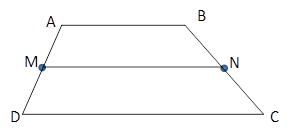Chapter 4.4, Problem 13E### Elementary Geometry for College St...

6th Edition
Daniel C. Alexander + 1 other
ISBN: 9781285195698

#### Solutions

Chapter
Section### Elementary Geometry for College St...

6th Edition
Daniel C. Alexander + 1 other
ISBN: 9781285195698
Textbook Problem
1 views

# In Exercises 11 to 16, the drawing shows trapezoid ABCD with A B ¯ | | D C ¯ ; also, M and N are midpoints of A D ¯ and B C ¯ , respectively.Exercises 11-16Given: A B = 8.2   a n d   M N = 9.5 Find: D C

To determine

To Find:

DC of trapezoid ABCD.

Explanation

Consider the following trapezoid ABCD.

Given:

AB=8.2 and MN=9.5

In the given trapezoid, AB¯||DC¯.

M is the midpoint of the side AD¯ and N is the midpoint of the side BC¯

### Still sussing out bartleby?

Check out a sample textbook solution.

See a sample solution

#### The Solution to Your Study Problems

Bartleby provides explanations to thousands of textbook problems written by our experts, many with advanced degrees!

Get Started

#### Solve the equations in Exercises 126. (x2+1)x+1(x+1)3=0

Finite Mathematics and Applied Calculus (MindTap Course List)

#### In Exercises 2340, find the indicated limit. 40. limx3xx2+72x2x+3

Applied Calculus for the Managerial, Life, and Social Sciences: A Brief Approach

#### Evaluate the integral 39. 01/2xe2x(1+2x)2dx

Single Variable Calculus: Early Transcendentals

#### The graph at the right is the direction field for: a) y = x y b) y = xy c) y = x + y d) y = xy

Study Guide for Stewart's Single Variable Calculus: Early Transcendentals, 8th